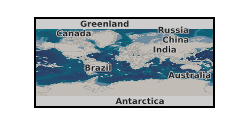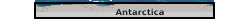Keyword

## Finite element analysis

5 record(s)

Type of resources
Topics
Keywords
Contact for the resource
Provided by
Years
Formats
Update frequencies
From 1 - 5 / 5
• # 3D GIA model output (van der Wal et al. 2015) (NERC grant NE/K009958/1)Numerical model predictions of present-day solid Earth deformation and gravity field change due to ongoing glacial isostatic adjustment processes. Model accounts for 3D spatial variations in Earth rheology using a finite element approach.

• # Geomechanical and discrete fracture networks in Thomas et al. 2020, Journal of Geophysical Research: Solid Earth (NERC Grant NE/L000660/1)Data supporting 'Effective permeability tensors of three-dimensional numerically grown geomechanical discrete fracture networks with evolving geometry and mechanical apertures', submitted to the Journal of Geophysical Research: Solid Earth. Authors: Robin N Thomas (corresponding, robin.thomas11@imperial.ac.uk), Adriana Paluszny, Robert W Zimmerman. Department of Earth Science and Engineering, Imperial College London. Contents: For each GDFN, the geometry at each growth step. Additionally, for GDFN E, the data shown in the paper (aperture and flow distributions, figures 6 and 7) are provided, including the displacement for the mechanical case, and pressure distributions which were not shown in the manuscript. For the two SDFN sets, the geometry of the four datasets shown in figures 4 and 5 are provided. Notes: - The geometry files are provided in the .3dm format, Rhinocerous' native format (https://www.rhino3d.com/). A free trial of Rhinocerous can be used to explore the files, and can convert them to a range of other CAD file types. - VTK files can be viewed using free software such as Paraview (https://www.paraview.org/). These contain the meshes. - Fracture surface areas reported in the paper are derived from the mesh, rather than the geometry. The mesh approximates the geometry leading to a different surface area than those measured in the geometry (3dm) files. - The SDFN datasets are shown before trimming the parts of fractures which are outside the domain. These parts are trimmed when they are imported to ICGT.

• # 3D GIA model output (King et al. 2016) (NERC grant NE/K009958/1)Numerical model predictions of present-day horizontal deformation due to ongoing glacial isostatic adjustment processes at GPS sites across Antarctica. Model accounts for 3D spatial variations in Earth rheology using a finite element approach.

• # Supporting data for 'DFENS: Diffusion chronometry using Finite Elements and Nested Sampling' (NERC Grant NE/L002507/1)This is supporting data for the manuscript entitled 'DFENS: Diffusion chronometry using Finite Elements and Nested Sampling' by E. J. F. Mutch, J. Maclennan, O. Shorttle, J. F. Rudge and D. Neave. Preprint here: https://doi.org/10.1002/essoar.10503709.1 Data Set S1. ds01.csv Electron probe microanalysis (EPMA) profile data of olivine crystals used in this study. Standard deviations are averaged values of standard deviations from counting statistics and repeat measurements of secondary standards. Data Set S2. ds02.csv Plagioclase compositional profiles used in this study, including SIMS, EPMA and step scan data. Standard deviations for EPMA analyses are averaged values of standard deviations from counting statistics and repeat measurements of secondary standards. Standard deviations for SIMS and step scan analyses are based on analytical precision of secondary standards. Data Set S3. ds03.csv Angles between the EPMA profile and the main olivine crystallographic axes measured by electron backscatter diffraction (EBSD). 'angle100X' is the angle between the  crystallographic axis and the x direction of the EBSD map, 'angle100Y' is the angle between  crystallographic axis and the y direction of the EBSD map, and 'angle100Z' is the angle between the  crystallographic axis and the z direction in the EBSD map etc. 'angle100P' is the angle between the EPMA profile and the  crystallographic axis, 'angle010P' is the angle between the EPMA profile and the  crystallographic axis, and 'angle100P' is the angle between the EPMA profile and the  crystallographic axis. All angles are in degrees. Data Set S4. ds04.csv Median timescales and 1 sigma errors from the olivine crystals of this study. The +1 sigma (days) is the quantile value calculated at 0.841 (i.e. 0.5 + (0.6826 / 2)). The -1 sigma (days) is therefore the quantile calculated at approximately 0.158 (which is 1 - 0.841). The 2 sigma is basically the same but it is 0.5 + (0.95/2). The value quoted as the +1 sigma (error) is the difference between the upper 1 sigma quantile and the median. Likewise the -1 sigma (error) is the difference between the median and the lower 1 sigma quantile. Data Set S5. ds05.xlsx Median timescales and 1 sigma errors from the plagioclase crystals of this study. Results from each of the parameterisations of the Mg-in-plagioclase diffusion data are included: Faak et al, (2013), Van Orman et al., (2014) and a combined expression. Data Set S6. ds06.xlsx Spreadsheet containing the regression parameters and covariance matrices used in this study and in Mutch et al. (2019). Additional versions of the olivine regressions where the ln fO2 is expressed in Pa have been made for completeness. We recommend using the versions where ln fO2 is expressed in its native form (bars).

• # Numerical modelling of fracture intensity increase due to interacting blast waves in three-dimensional granitic rocksThis work presents a detailed three-dimensional finite element based model for wave propagation, combined with a postprocessing procedure to determine the fracture intensity caused by blasting. The data generated during this project includes output files of all simulations with detailed fields, geometries and meshes. The model incorporates the Johnson-Holmquist-2 constitutive model, which is designed for brittle materials undergoing high strain rates and high pressures and fracturing, and a tensile failure model. Material heterogeneity is introduced into the model through variation of the material properties at the element level, ensuring jumps in strain. The algorithm for the combined Johnson-Holmquist-2 and tensile failure model is presented and is demonstrated to be energy-conserving, with an open-source MATLABTM implementation of the model. A range of sub-scale numerical experiments are performed to validate the modelling and postprocessing procedures, and a range of materials, explosive waves and geometries are considered to demonstrate the model's predictive capability quantitatively and qualitatively for fracture intensity. Fracture intensities on 2D planes and 3D volumes are presented. The mesh dependence of the method is explored, demonstrating that mesh density changes maintain similar results and improve with increasing mesh quality. Damage patterns in simulations are self-organising, forming thin, planar, fracture-like structures that closely match the observed fractures in the experiments. The presented model is an advancement in realism for continuum modelling of blasts as it enables fully three-dimensional wave interaction, handles damage due to both compression and tension, and relies only on measurable material properties. The uploaded data are the specific simulation outputs for four explosion models occurring on two different rock types, and the specific fracture patterns generated.